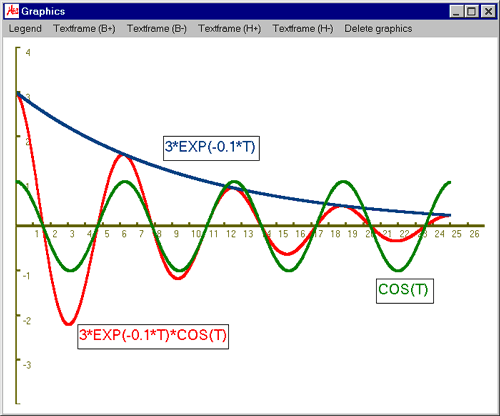# MasterAllRound: the PC calculator

## Plot module: example damped vibration

This example shows how to print the graph of the damped vibration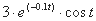and its component parts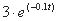and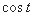This is the input for MasterAllRound: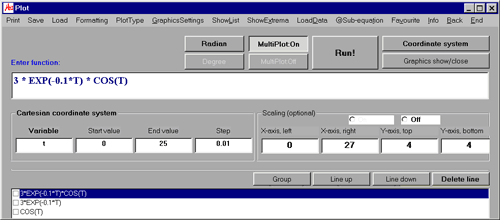• Activate "Radian" by clicking on the button
• The variable is "t". The graph of the function is to be plotted from "0" till "25" with a step width of "0.01":
• Variable: t
• Start value: 0
• End value: 25
• Step width: 0.01
• Scaling:
• X-axis, left: 0
• X-axis, right: 27
• Y-axis, top: 4
• Y-axis, bottom: 4
• Input of the function:
• 3*EXP(-0.1*T)*COS(T)
• Activate "Multiplot: On" by clicking on the button and enter the first part of the equation:
• 3*EXP(-0.1*T)
• Do the same with the second part of the equation:
• COS(T)